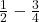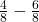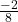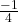## Can the exponent in the denominator be subtracted from the exponent in the numerator when the based are different? Explain

Question

Can the exponent in the denominator be subtracted from the exponent in the numerator when the based are different? Explain

in progress 0
6 months 2021-07-24T19:21:42+00:00 1 Answers 3 views 0

## Answers ( )

No, Sorry.

Step-by-step explanation:

Since the bases are different, you’ll have to change them even if they have a exponent or not. If you dunno how to make the denominators the same, just multiply the two by eachother.

Example) 1/2 – 3/4Multiply 2 and 4 together, and then multiply the 4 to the one also. Multiply 2 to four and also to the 3.The subtract from there.and then Reduce (if needed.)